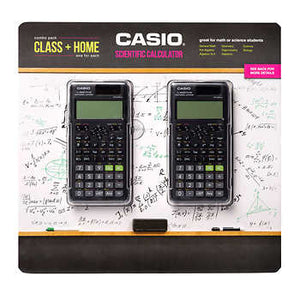With just one click we ship you all what you need!English en
•English en
•Español es
With just one click we ship you all what you need!English en
•English en
•Español es
Cart 0# Casio FX-300ESPLS2-S 2nd Edition Scientific Calculator, 2-pack

Regular price \$17.99 \$0.00 Unit price per
Shipping calculated at checkout.
Casio FX-300ESPLS2-S 2nd Edition Scientific Calculator with sleek new design and slide on hard case with Natural Textbook Display and improved math functionality. It has been designed as the perfect choice for middle school through high school students learning General Math, Trigonometry, Statistics, Algebra I and II, Pre-Algebra, Geometry, Physics.

• Natural Textbook Display- Can display fractions, derivatives, integrals, sum and other operations similar to their display in math textbooks.
• Statistics and Linear Regression: This calculator can linear regression, quadratic regression, logarithmic regression, e exponential regression, ab exponential regression, power regression and inverse regression.
• Converts between Standard form and a Decimal: This calculator can transform a displayed decimal calculation result to a fraction or mixed number. It can also be converted back to the decimal form.
• Review/edit previous entries: Allow you to scroll up on the screen to see and edit previously made calculations.
• 2-function Table of values [f(x), g(x)]: The Table mode allows you to input a function and view the table of values for that function.
• Prime Factorization: Allows you to determine the set of prime numbers which multiply together to get the original integer.
• Random Number Generator: Allows you to randomly generate integers.
• GCD/LCM: Allows you to find the Greatest Common Divisor and Least Common Multiple of 2 or more integers.
• Integer Division with a Remainder: Allows the option to divide integers and get an answer as a remainder rather than in decimal form.
• 249 Built-in Math Functions: Including basic and advanced scientific, exponential and trigonometric, fractions, regression analysis and more.
• Operating system: Entry Logic: V.P.A.M.
• Greatest Common Divisor, Least Common Multiple

Accessories:
• Slide-On Hard Protective Case

Dimensions:
• W 3 1/8 x L 6 3/8 x H ½ inches 3.7 oz.